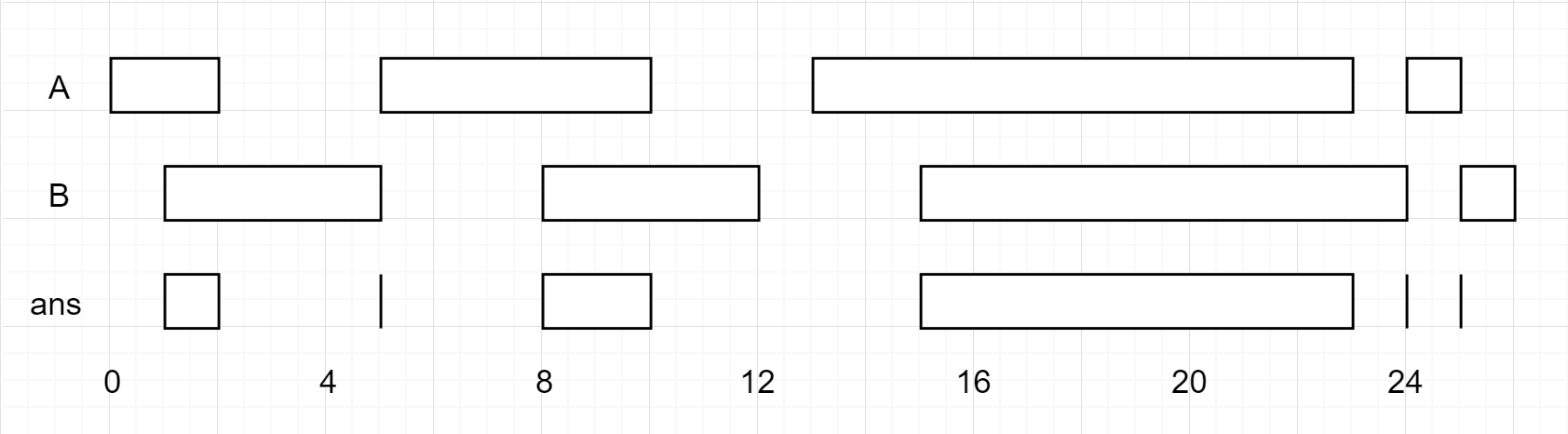Amazon

Apple

Oracle

Snapchat

Uber

2020-05-23

## 986. Interval List Intersections

### Question:

Given two lists of closed intervals, each list of intervals is pairwise disjoint and in sorted order.

Return the intersection of these two interval lists.

(Formally, a closed interval `[a, b]` (with `a <= b`) denotes the set of real numbers `x` with `a <= x <= b`.  The intersection of two closed intervals is a set of real numbers that is either empty, or can be represented as a closed interval.  For example, the intersection of [1, 3] and [2, 4] is [2, 3].)

#### Example 1:``````Input: A = [[0,2],[5,10],[13,23],[24,25]], B = [[1,5],[8,12],[15,24],[25,26]]
Output: [[1,2],[5,5],[8,10],[15,23],[24,24],[25,25]]
Reminder: The inputs and the desired output are lists of Interval objects, and not arrays or lists.
``````

### Solution:

Using two pointers, one for the array A and one for the B. If current position of two arrays have intersection, add to the result list. Otherwise, move the array with smallest ending value to next position. The intesection is the maximum value on the start side and minimum value on the end side.

``````class Solution {
public int[][] intervalIntersection(int[][] A, int[][] B) {
List<int[]> result = new ArrayList<>();

if (A == null | B == null | A.length == 0 | B.length == 0) return new int[][]{};

int A_pointer = 0, B_pointer = 0;

while(A_pointer < A.length && B_pointer < B.length) {
int start = Math.max(A[A_pointer], B[B_pointer]);
int end = Math.min(A[A_pointer], B[B_pointer]);

if (start <= end) {